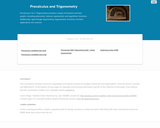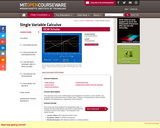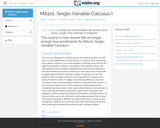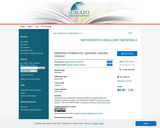Updating search results...

# 44 Results

View
Selected filters:
• CalculusRating
0.0 stars

Precalculus 1 & 2 / Trigonometry provides a study of functions and their graphs, including polynomial, rational, exponential, and logarithmic functions. Additionally, right-triangle trigonometry, trigonometric functions and their applications are covered.

Subject:
Calculus
Mathematics
Trigonometry
Material Type:
Full Course
Textbook
Provider:
Lumen Learning
Provider Set:
Candela Courseware
Author:
David Lippman
Melonie Rasmussen
03/04/2019Conditional Remix & Share Permitted
CC BY-NC-SA
Rating
0.0 stars

This calculus course covers differentiation and integration of functions of one variable, and concludes with a brief discussion of infinite series. Calculus is fundamental to many scientific disciplines including physics, engineering, and economics.

Subject:
Calculus
Mathematics
Material Type:
Full Course
Provider:
M.I.T.
Provider Set:
M.I.T. OpenCourseWare
Author:
Jerison, David
01/01/2010Unrestricted Use
CC BY
Rating
0.0 stars

This course is designed to introduce the student to the study of Calculus through concrete applications. Upon successful completion of this course, students will be able to: Define and identify functions; Define and identify the domain, range, and graph of a function; Define and identify one-to-one, onto, and linear functions; Analyze and graph transformations of functions, such as shifts and dilations, and compositions of functions; Characterize, compute, and graph inverse functions; Graph and describe exponential and logarithmic functions; Define and calculate limits and one-sided limits; Identify vertical asymptotes; Define continuity and determine whether a function is continuous; State and apply the Intermediate Value Theorem; State the Squeeze Theorem and use it to calculate limits; Calculate limits at infinity and identify horizontal asymptotes; Calculate limits of rational and radical functions; State the epsilon-delta definition of a limit and use it in simple situations to show a limit exists; Draw a diagram to explain the tangent-line problem; State several different versions of the limit definition of the derivative, and use multiple notations for the derivative; Understand the derivative as a rate of change, and give some examples of its application, such as velocity; Calculate simple derivatives using the limit definition; Use the power, product, quotient, and chain rules to calculate derivatives; Use implicit differentiation to find derivatives; Find derivatives of inverse functions; Find derivatives of trigonometric, exponential, logarithmic, and inverse trigonometric functions; Solve problems involving rectilinear motion using derivatives; Solve problems involving related rates; Define local and absolute extrema; Use critical points to find local extrema; Use the first and second derivative tests to find intervals of increase and decrease and to find information about concavity and inflection points; Sketch functions using information from the first and second derivative tests; Use the first and second derivative tests to solve optimization (maximum/minimum value) problems; State and apply Rolle's Theorem and the Mean Value Theorem; Explain the meaning of linear approximations and differentials with a sketch; Use linear approximation to solve problems in applications; State and apply L'Hopital's Rule for indeterminate forms; Explain Newton's method using an illustration; Execute several steps of Newton's method and use it to approximate solutions to a root-finding problem; Define antiderivatives and the indefinite integral; State the properties of the indefinite integral; Relate the definite integral to the initial value problem and the area problem; Set up and calculate a Riemann sum; Estimate the area under a curve numerically using the Midpoint Rule; State the Fundamental Theorem of Calculus and use it to calculate definite integrals; State and apply basic properties of the definite integral; Use substitution to compute definite integrals. (Mathematics 101; See also: Biology 103, Chemistry 003, Computer Science 103, Economics 103, Mechanical Engineering 001)

Subject:
Calculus
Mathematics
Material Type:
Assessment
Full Course
Homework/Assignment
Syllabus
Textbook
Provider:
The Saylor Foundation
03/04/2019Unrestricted Use
CC BY
Rating
0.0 stars

These open-source mathematics homework problems are programmed for the WeBWorK mathematics platform and correspond to chapters in OpenStax Calculus Volume I. They were created through a Round Eight Textbook Transformation Grant.

Subject:
Calculus
Mathematics
Material Type:
Interactive
Provider:
University System of Georgia
Provider Set:
Galileo Open Learning Materials
Author:
Scott Kersey
Stephen Carden